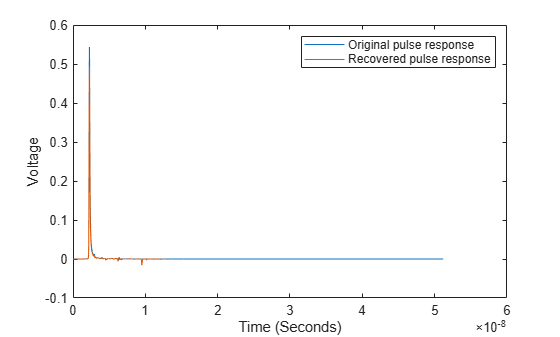# wave2pulse

Pulse response from data pattern waveform

## Syntax

``P = wave2pulse(W,D,N)``
``P = wave2pulse(___,K)``

## Description

example

````P = wave2pulse(W,D,N)` converts a data pattern waveform `W` to a pulse response `P`, given the symbol pattern `D` and the number of samples per symbol `N`.```
````P = wave2pulse(___,K)` converts a data pattern waveform to a truncated pulse response so that the first dimension of `P` is of size (`N`·`K`), where `K` is the desired length of the pulse response.```

## Examples

collapse all

Load pulse response column matrix from a file.

`load('PulseResponseReflective100ps.mat');`

Select the primary pulse response and remove any DC components.

`P1 = pulse(:,1) - pulse(1,1);`

Create a symbol pattern of PRBS order 7.

```order = 7; data = prbs(order,2^order-1)-0.5;```

Create a data pattern waveform from the pulse response.

`W1 = pulse2wave(P1,data,SamplesPerSymbol); `

Apply memoryless nonlinearity to the waveform using a `serdes.SaturatingAmplifier` object. The saturating amplifier clips any voltage over `0.4` V.

```SatAmp = serdes.SaturatingAmplifier('Limit',0.4); W2 = SatAmp(W1);```

Recover the pulse response from the waveform `W2`.

`P2 = wave2pulse(W2,data,SamplesPerSymbol);`

Plot the original and recovered pulse responses.

```t2 = dt*(0:length(P2)-1); figure plot(t,P1,t2,P2) xlabel('Time (Seconds)') ylabel('Voltage') legend('Original pulse response','Recovered pulse response')```## Input Arguments

collapse all

Data pattern waveform, specified as a column vector.

Data Types: `double`

Symbol pattern contained within the data pattern waveform `W`, specified as a vector.

Data Types: `double`

Number of samples per symbol, specified as a positive integer scalar.

Data Types: `double`

Desired length of the pulse response, specified as a positive integer scalar in symbols.

Data Types: `double`

## Output Arguments

collapse all

Converted pulse response, returned as a column matrix. The first column contains the primary pulse response and the subsequent columns (if any) contain the crosstalk pulse responses.

Data Types: `double`

## Version History

Introduced in R2020a